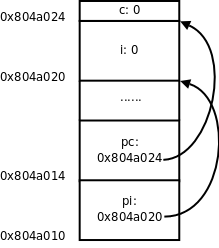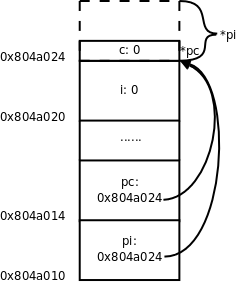## 1. 指针的基本概念

```int i;
int *pi = &i;
char c;
char *pc = &c;````int *p, *q;`

`pi = &j;`

`*pi = *pi + 10;`

`&`运算符的操作数必须是左值，因为只有左值才表示一个内存单元，才会有地址，运算结果是指针类型。`*`运算符的操作数必须是指针类型，运算结果可以做左值。所以，如果表达式`E`可以做左值，`*&E``E`等价，如果表达式`E`是指针类型，`&*E``E`等价。

`int *ptri = pi;`

```int *ptri;
ptri = pi;```

`pi = (int *)pc;````int main(void)
{
int *p;
...
*p = 0;
...
}```

```int main(void)
{
int *p = NULL;
...
*p = 0;
...
}```

`NULL`在C标准库的头文件`stddef.h`中定义：

`#define NULL ((void *)0)`

```void func(void *pv)
{
/* *pv = 'A' is illegal */
char *pchar = pv;
*pchar = 'A';
}

int main(void)
{
char c;
func(&c);
printf("%c\n", c);
...
}```